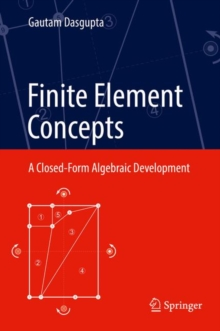Supporting your high street Find out how »
• My Account# Finite Element Concepts : A Closed-Form Algebraic Development Hardback

#### Description

This text presents a highly original treatment of the fundamentals of FEM, developed using computer algebra, based on undergraduate-level engineering mathematics and the mechanics of solids.

The book is divided into two distinct parts of nine chapters and seven appendices.

The first chapter reviews the energy concepts in structural mechanics with bar problems, which is continued in the next chapter for truss analysis using Mathematica programs.

The Courant and Clough triangular elements for scalar potentials and linear elasticity are covered in chapters three and four, followed by four-node elements.

Chapters five and six describe Taig's isoparametric interpolants and Iron's patch test.

Rayleigh vector modes, which satisfy point-wise equilibrium, are elaborated on in chapter seven along with successful patch tests in the physical (x,y) Cartesian frame.

Chapter eight explains point-wise incompressibility and employs (Moore-Penrose) inversion of rectangular matrices.

The final chapter analyzes patch-tests in all directions and introduces five-node elements for linear stresses.

Curved boundaries and higher order stresses are addressed in closed algebraic form.

Appendices give a short introduction to Mathematica, followed by truss analysis using symbolic codes that could be used in all FEM problems to assemble element matrices and solve for all unknowns.

All Mathematica codes for theoretical formulations and graphics are included with extensive numerical examples.

#### Information

• Format: Hardback
• Pages: 333 pages, 45 Illustrations, black and white; XXXVI, 333 p. 45 illus.
• Publisher: Springer-Verlag New York Inc.
• Publication Date:
• Category: Differential calculus & equations
• ISBN: 9781493974214

#### Other Formats

£79.99

£65.65

on all orders

###### Pick up orders

from local bookshops

£79.50

£67.58

£79.99

£65.65